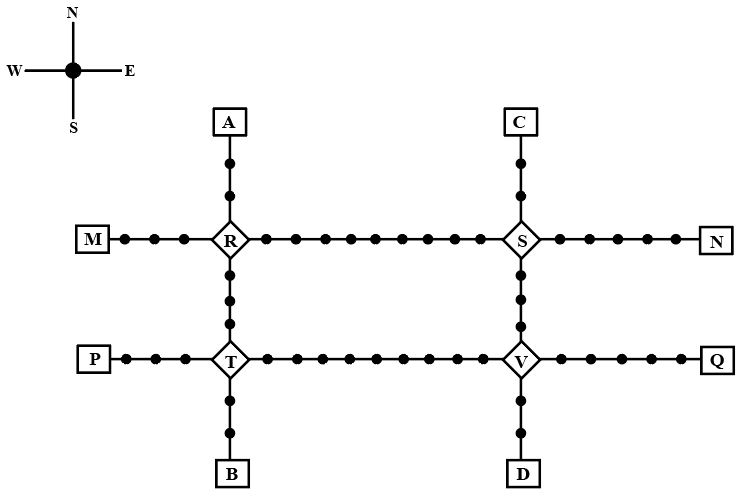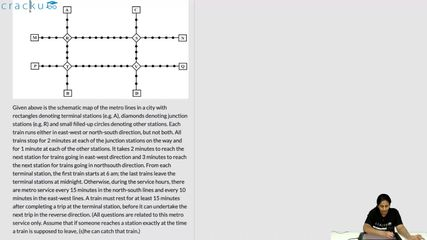### CAT 2022 Question Paper (Slot 1) Question 41

InstructionsGiven above is the schematic map of the metro lines in a city with rectangles denoting terminal stations (e.g. A), diamonds denoting junction stations (e.g. R) and small filled-up circles denoting other stations. Each train runs either in east-west or north-south direction, but not both. All trains stop for 2 minutes at each of the junction stations on the way and for 1 minute at each of the other stations. It takes 2 minutes to reach the next station for trains going in east-west direction and 3 minutes to reach the next station for trains going in northsouth direction. From each terminal station, the first train starts at 6 am; the last trains leave the terminal stations at midnight. Otherwise, during the service hours, there are metro service every 15 minutes in the north-south lines and every 10 minutes in the east-west lines. A train must rest for at least 15 minutes after completing a trip at the terminal station, before it can undertake the next trip in the reverse direction. (All questions are related to this metro service only. Assume that if someone reaches a station exactly at the time a train is supposed to leave, (s)he can catch that train.)

Question 41

# If Priya is ready to board a train at 10:25 am from station T, then when is the earliest that she can reach station S?

Solution

Priya can reach S from T via R or V.

In the east-west direction, the first train from P arrives at T at time = 6 am + $$\left(4\times2\right)+\left(3\times1\right)=\ 11$$ minutes = 6:11 am

Since T is a junction so this train will halt for 2 minutes at T and leave at 6:13.

Since every 10 minutes, a train starts from P in the east-west direction so the latest by which Priya will be able to board such a train is at 10:33 am.

In the north-south direction, the first train from B arrives at T at time = 6 am + $$\left(3\times3\right)+\left(2\times1\right)=\ 11$$ minutes = 6:11 am

Since T is a junction so this train will halt for 2 minutes at T and leave at 6:13.

Since every 15 minutes a train starts from P in the east-west direction so the latest by which Priya will be able to board such a train is at 10:28 am.

Now since she will be able to board a north-south train earlier than the east-west train so Priya will board a train for R from T at 10:28 am.

There are 3 stations between T and R

Travelling time between T and R = $$\left(4\times3\right)+\left(\left(3\times1\right)\right)=\ 15$$ minutes

Therefore, Priya will reach R latest by 10:43 am

In the east-west direction, the first train from M arrives at R at time = 6 am + $$\left(4\times2\right)+\left(3\times1\right)=\ 11$$ minutes = 6:11 am

Since R is a junction so this train will halt for 2 minutes at R and leave at 6:13.

Since every 10 minutes, a train starts from M in the east-west direction, so the latest by which Priya will be able to board such a train is at 10:43 am.

There are 9 stations between R and S

Travelling time between R and S = $$\left(10\times2\right)+\left(9\times1\right)=29\$$ minutes

Time by which she reaches S = 10:43 +29 minutes = 11:12 am

If Priya boards a east-west train then Priya will board a train for V from T at 10:33 am.

There are 9 stations between T and V

Travelling time between T and V = $$\left(10\times2\right)+\left(9\times1\right)=29\$$ minutes

Therefore, Priya will reach V latest by 10:33 am + 29 minutes = 11:02 am

In the north-south direction, the first train from D arrives at V at time = 6 am + $$\left(3\times3\right)+\left(2\times1\right)=\ 11$$ minutes = 6:11 am

Since V is a junction so this train will halt for 2 minutes at V and leave at 6:13.

Since every 15 minutes, a train starts from D in the north-south direction, so the latest by which Priya will be able to board such a train from V is at 11:13 am.

There are 3 stations between V and S

Travelling time between R and S =$$\left(4\times3\right)+\left(\left(3\times1\right)\right)=\ 15$$ minutes

Time by which she reaches S = 11:13 +15 minutes = 11:28 am

### View Video Solution• All Quant CAT Formulas and shortcuts PDF
• 30+ CAT previous papers with solutions PDF